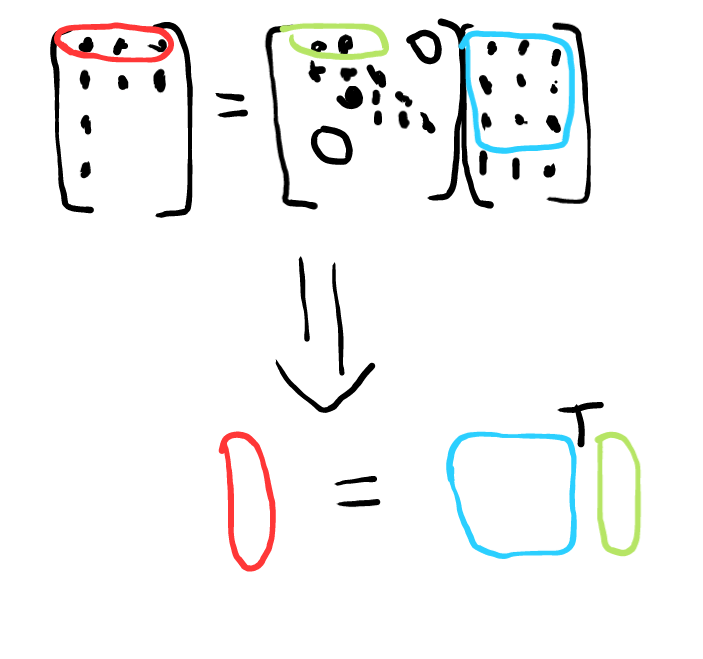So as part of betting the banded hessian out of pytorch, I’ve been trying to think about what it is I am doing and I’ve come up with an alternative way of talking about it.

If every row of a matrix has <N non zero entries, you can back out that matrix from N matrix vector samples of it. You have many choices for the possible sampling vectors. Random works well.The unknown in this case is the row of the matrix, represented in green. We put a known set of inputs into it and get outputs. Each row of the output, represented in red, can tell use the matrix row. We have to invert the matrix that consists of all the elements that the nonzero elements of that row touches represented in blue. That black T is a transpose. To turn those row vectors into column vectors, we have to transpose everything.

For a banded matrix, we have a shifting sample matrix that we have to invert, one for each row.

import numpy as np
from scipy.linalg import toeplitz as toep
N =10
bandn = 3

row = np.zeros(N)
row = -2
row = 1

banded = toep(row) #np.eye(N)
print(banded)
samples = np.random.randn(N,bandn)
print(samples)
y = banded @ samples
print(y)

band = np.zeros((N,bandn))

circsamples = np.random.randn(N+bandn,bandn)
circsamples[bandn//2:-bandn//2, :] = samples

for j in range(N):
band[j,:] = np.linalg.solve(circsamples[j:j+bandn, :].T,  y[j,:])
print(band)
'''
for j in range(N-bandn):
band[j+bandn//2,:] = np.linalg.solve(samples[j:j+bandn, :].T,  y[j+bandn//2,:])
'''

#corners

'''
for j in range(bandn//2):
band[j,j+bandn//2:] = np.linalg.solve(samples[0:j+bandn//2+1, 0:j+bandn//2+1].T,  y[j,:j+bandn//2+1])
'''

#print(band)


A cute trick we can use to simplify the edge cases where we run off the ends is to extend the sample matrix with some random trash. That will actually put the entries in the appropriate place and will keep the don’t cares close to zero also.

In my previous post I used a stack of identity matrices. These are nice because a shifted identity matrix is a circular permutation of the entries, which is very easy to undo. That was what the loop that used numpy.roll was doing. Even better, it is easy to at least somewhat vectorize the operation and you can produce those sampling vectors using some clever use of broadcasting of an identity matrix.

An alternative formulation that is a little tighter. I want the previous version because the samples isn’t actually always random. Often they won’t really be under our control.

import numpy as np
from scipy.linalg import toeplitz as toep
N =10
bandn = 3

row = np.zeros(N)
row = -2
row = 1

banded = toep(row) #np.eye(N)
print(banded)
samples = np.random.randn(N,bandn)
print(samples)
y = banded @ samples
print(y)

band = np.zeros((N,bandn))

circsamples = np.random.randn(N+bandn,bandn)
circsamples[bandn//2:-bandn//2, :] = samples

for j in range(N):
band[j,:] = np.linalg.solve(circsamples[j:j+bandn, :].T,  y[j,:])
print(band)


This all still doesn’t address the hermitian problem. The constraint that A is hermitian hypothetically allows you to take about half the samples. But the constraints make it tougher to solve. I haven’t come up with anything all that much better than vectorizing the matrix and building a matrix out of the samples in the appropriate spots.

I think such a matrix will be banded if A is banded, so that’s something at least.